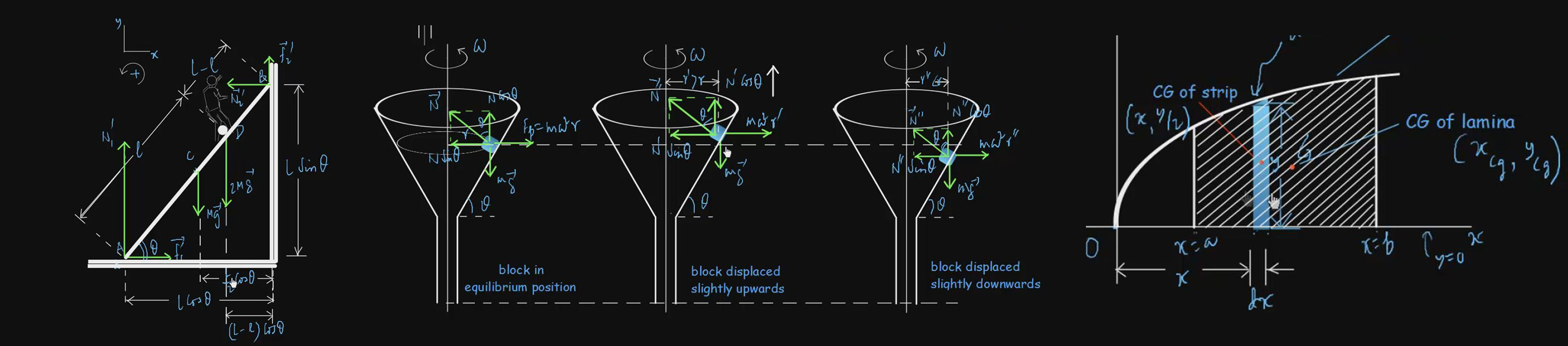# Newton's Laws of Motion

Newton’s three laws of motion are fundamental to the study of Kinetics. Kinetics is the study of how motion of a body is related to its mass and the force(s) acting on it. The force represents the interaction of a body with its environment. In general, the environment consists of nearby bodies and the effect of distant bodies may be ignored. The mass of a body is a measure of its inertia which is the tendency to resist acceleration under a force. The theory of motion was developed by English physicist Sir Isaac Newton (1642 – 1727) in the 17th century. We have video lectures on various subtopics such as Newton’s Laws of Motion, Concept of Force, Free Body Diagrams, Applications of Newton’s Laws, Pseudo Force, Comparison of Inertial Frame And Non-Inertial Frame etc. to provide an in-depth knowledge in an extremely skillful way.

#### Basic level videos

Problems On Application Of Newtons Laws Part 50:10 Basic
300 5
Problems On Application Of Newtons Laws Part II 1:00:17 Basic
300 5
Comparison Of Intertial-Frame And Non-Intertial-Frame Methods 46:49 Basic
300 5
Concept Of Force 1:12:00 Basic
350 7.5
Constrained Motion Of Pulley-Block System 1:37:07 Basic
450 8
Constrained Motion Of Wedge-Block System 37:10 Basic
200 3
Free Body Diagram 1:00:37 Basic
300 5
Newton's First Law Of Motion I 48:50 Basic
300 5
Newton's First Law Of Motion II 30:17 Basic
200 3
Newton's Second Law Of Motion 1:15:50 Basic
350 7.5
Newton's Third Law Of Motion And Units Of Force 58:18 Basic
300 5
Pseudo Force With Examples 1:08:44 Basic
300 5
Study Of Wedge-Block System By Pseudo-Force Method 44:45 Basic
250 4
Application Of Newton's Law 1:02:48 Basic
350 7.5
Applications Of Newton's Laws II 38:17 Basic
200 3
Problems On Pulleys And Wedge-block System 36:09 Basic
200 3
Problems On Pulleys And Wedge-block System II 54:21 Basic
300 5

#### Advanced level Videos Note: (CE) Stands for Problems from Competitive Examination Papers

Advanced-Level Problems On Newton's Laws Of Motion I 1:09:36
350
7.5
Advanced-Level Problems On Newton's Laws Of Motion II 52:25
300
5
Advanced-Level Problems On Newton's Laws Of Motion III 1:08:15
350
7.5
Advanced-Level Problems On Newton's Laws Of Motion IV 1:23:28
350
7.5
Advanced-level Problems On Newton's Laws Of Motion V 1:11:53
350
7.5
Problems On Newton's Laws Of Motion (CE) 1:08:48
350
7.5